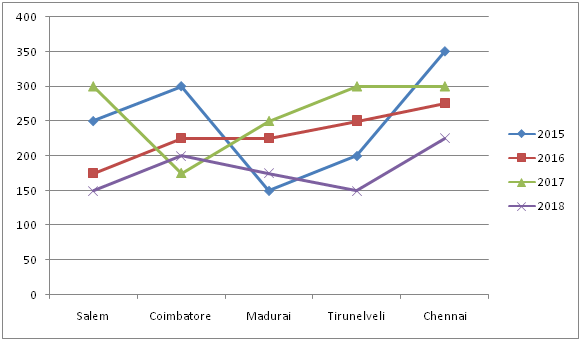# gkrecall

We brings you daily Current affairs, daily Current Affairs Quiz, weekly Current Affairs, weekly Current Affairs Quiz to enhance your preparation for upcoming exams. We also provide articles related to quant, reasoning, English, ssc etc .

## QUANT PRACTICE SET 11 FOR RRB PO & CLERK PRE-2020

RRB Clerk and RRB PO Prelims exam are going to be held in the upcoming months. We have already provided you with the PDFs of many topics of Quantitative Aptitude like Simplification/Approximation, Number Series,datainterpretation.important Arithmetic questions to prepare for RRB Clerk and PO Pre exam. Practicing these questions will help you to know about the level of the questions. To increase your speed and accuracy, enhance your calculations.

### START QUIZ

Directions (1 – 5): Following question contains two equations as I and II. You have to solve both equations and determine the relationship between them.
1)
I) x2 -22x + 96 =0
II) y2 -20y + 91 =0
a) x < y
b) x ≥ y
c) x = y or relationship cannot be determined.
d) x > y
e) x ≤ y
2)
I) x2 = 1089
II) y = √1369
a) x ≥ y
b) x ≤ y
c) x = y or relationship cannot be determined.
d) x> y
e) x< y
3)
I) x2–x – 272=0
II) y2 +y- 156 =0
a) x = y or relationship cannot be determined.
b) x ≥ y
c) x< y
d) x> y
e) x ≤ y
4)
I) 5x2 -18x +9 =0
II) 20y2 -13y + 2 =0
a) x = y or relationship cannot be determined.
b) x ≥ y
c) x> y
d) x< y
e) x ≤ y
5)
I) 2x2 -x -1 =0
II) 2y2 -4y + 2 =0
a) x = y or relationship cannot be determined.
b) x ≥ y
c) x> y
d) x< y
e) x ≤ y
Directions (6 – 10): Study the following information carefully and answer the questions given below.
Number of persons/candidates selected in SBI PO Examination from different cities in the year 2015 to 2018.6) What is the difference between number of persons selected from Salem and number of persons selected from Chennai in all the years together?
a) 225
b) 275
c) 325
d) 250
e) 280
7) The ratio between numbers of boys to number of girls selected from Madurai in 2017 is 2:3.The number of girls selected from Madurai in 2017 is 75 less than number of girls selected from Chennai. What is the number of boys selected from Chennai in 2017?
a) 75
b) 95
c) 110
d) 125
e) Cannot be determined
8) Total number of candidates selected from all the cities in the year 2018 is what percent of total number of candidate selected from all the cities in 2016?
a) 66 (2/3) %
b) 82 (1/3) %
c) 70.5 %
d) 78.2 %
e) 63.8 %
9) What is the respective ratio between total number of candidate selected from Tirunelveli in 2015 & 2018 to total number of candidate selected from Coimbatore in 2016 &2017?
a) 4: 5
b) 2: 3
c) 7: 8
d) 6: 7
e) 5: 6
10) The number of candidate selected from Tirunelveli in the year 2014 is 42 % of candidate selected from Tirunelveli in 2015. The ratio between number of girls to boys selected from Tirunelveli in 2014 is 7: 5. What is the number of girls selected from Tirunelveli in 2014?
a) 56
b) 49
c) 30
d) 42
e) 35
SOLUTION

Direction (1-5) :
x2 -22x + 96 =0
x2 -16x -6x + 96 =0
(x-16) (x-6) =0
=>x = 16, 6
y2 -20y + 91 =0
y2 -13y- 7y + 91 =0
(y-13) (y-7) =0
=>y= 13, 7
Hence the relationship cannot be determined.
I) x2 = 1089
x = 33,-33
II) y = √1369
y = 37
Hence x < y
I) x2 -x -272 =0
x2 -17x+ 16x – 272 =0
(x-17) (x+16) =0
x = 17, -16
II) y2 +y -156 =0
y2 +13y- 12y – 156 =0
(y+13) (y-12) =0
y = -13, 12
Hence the relationship cannot be determined.
I) 5x2 -18x + 9 =0
5x2 -15x-3x +9 =0
(5x -3) (x-3) = 0
x = 3, 3/5
II) 20y2 -13y + 2 =0
20y2 -8y- 5y + 2 =0
(5y -2) (4y-1) = 0
y = 2/5, 1/4
Hence x > y
I) 2x2 -x -1 =0
2x2 -2x +x-1 =0
(2x +1) (x-1) = 0
x = -1/2, 1
II) 2y2 -4y + 2 =0
2y2 -2y-2y + 2 =0
(2y -2) (y-1) = 0
y = 1, 1
Hence x ≤ y
Direction (6-10) :
Number of persons selected from Salem =150 +175+ 250 +300 = 875
Number of persons selected from Chennai = 225 +275 +300 +350 = 1150
Difference = 1150 -875 =275
Number of persons selected from Madurai in 2017 = 250
Ratio between number of boys to number of girls selected from Madurai in 2017 = 2:3
Number of boys selected from Madurai in 2017 =100
Number of girls selected from Madurai in 2017 =150
Number of girls selected from Chennai in 2017 =150 + 75 =225
Number of person selected from Chennai in 2017 = 300
Number of boys selected from Chennai in 2017 = 300 – 225 = 75
Total number of candidates selected from all the cities in 2018
= 150 + 200 + 175 + 150 + 225
= 900
Total number of candidate selected from all the cities in 2016
= 175 + 225 + 225 +250 + 275
= 1150
Required Percentage = (900/1150)×100 = 78.2 %